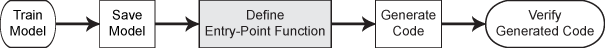Documentation

### This is machine translation

Mouseover text to see original. Click the button below to return to the English version of the page.

To view all translated materials including this page, select Country from the country navigator on the bottom of this page.

Reconstruct model object from saved model for code generation

To generate C/C++ code for the object functions (`predict`, `random`, `knnsearch`, or `rangesearch`) of machine learning models, use `saveCompactModel`, `loadCompactModel`, and `codegen`. After training a machine learning model, save the model by using `saveCompactModel`. Define an entry-point function that loads the model by using `loadCompactModel` and calls an object function. Then use `codegen` or the MATLAB® Coder™ app to generate C/C++ code. Generating C/C++ code requires MATLAB Coder.

This flow chart shows the code generation workflow for the object functions of machine learning models. Use `loadCompactModel` for the highlighted step.## Syntax

``Mdl = loadCompactModel(filename)``

## Description

example

````Mdl = loadCompactModel(filename)` reconstructs a classification model, regression model, or nearest neighbor searcher (`Mdl`) from the saved model stored in the MATLAB formatted binary file (MAT-file) named `filename`. You must create the `filename` file by using `saveCompactModel`.```

## Examples

collapse all

After training a machine learning model, save the model by using `saveCompactModel`. Define an entry-point function that loads the model by using `loadCompactModel` and calls the `predict` function of the trained model. Then use `codegen` to generate C/C++ code.

This example briefly explains the code generation workflow for the prediction of machine learning models at the command line. For more details, see Code Generation for Prediction of Machine Learning Model at Command Line. You can also generate code using the MATLAB Coder app. See Code Generation for Prediction of Machine Learning Model Using MATLAB Coder App for details. To learn about the code generation for finding nearest neighbors using a nearest neighbor searcher model, see Code Generation for Nearest Neighbor Searcher.

Train Model

Load Fisher's iris data set. Remove all observed setosa irises data so that `X` and `Y` contain data for two classes only.

```load fisheriris inds = ~strcmp(species,'setosa'); X = meas(inds,:); Y = species(inds);```

Train a support vector machine (SVM) classification model using the processed data set.

`Mdl = fitcsvm(X,Y);`

`Mdl` is a `ClassificationSVM` model.

Save Model

Save the SVM classification model to the file `SVMIris.mat` by using `saveCompactModel`.

`saveCompactModel(Mdl,'SVMIris');`

Define Entry-Point Function

Define an entry-point function named `classifyIrises` that does the following:

• Accept iris flower measurements with columns corresponding to `meas`, and return predicted labels.

• Load a trained SVM classification model.

• Predict labels using the loaded classification model for the iris flower measurements.

`type classifyIrises.m % Display contents of classifyIrises.m file`
```function label = classifyIrises(X) %#codegen %CLASSIFYIRISES Classify iris species using SVM Model % CLASSIFYIRISES classifies the iris flower measurements in X using the % compact SVM model in the file SVMIris.mat, and then returns class % labels in label. CompactMdl = loadCompactModel('SVMIris'); label = predict(CompactMdl,X); end ```

Add the `%#codegen` compiler directive (or pragma) to the entry-point function after the function signature to indicate that you intend to generate code for the MATLAB algorithm. Adding this directive instructs the MATLAB Code Analyzer to help you diagnose and fix violations that would result in errors during code generation.

Note: If you click the button located in the upper-right section of this example and open this example in MATLAB®, then MATLAB® opens the example folder. This folder includes the entry-point function file.

Generate Code

Generate code for the entry-point function using `codegen`. Because C and C++ are statically typed languages, you must determine the properties of all variables in the entry-point function at compile time. Pass `X` as the value of the `-args` option to specify that the generated code must accept an input that has the same data type and array size as the training data `X`. If the number of observations is unknown at compile time, you can also specify the input as variable-size by using `coder.typeof`. For details, see Specify Variable-Size Arguments for Code Generation and Specify Properties of Entry-Point Function Inputs (MATLAB Coder).

`codegen classifyIrises -args {X}`

`codegen` generates the MEX function `classifyIrises_mex` with a platform-dependent extension.

Verify Generated Code

Compare the labels classified using `predict`, `classifyIrises`, and `classifyIrises_mex`.

```label1 = predict(Mdl,X); label2 = classifyIrises(X); label3 = classifyIrises_mex(X); verify_label = isequal(label1,label2,label3)```
```verify_label = logical 1 ```

`isequal` returns logical 1 (true), which means all the inputs are equal. The labels classified all three ways are the same.

## Input Arguments

collapse all

Name of MAT-file containing the structure array representing a model object, specified as a character vector. You must create the `filename` file using `saveCompactModel`.

`loadCompactModel` reconstructs the model stored in the `filename` file at compile time. For supported models, see the `Mdl` input argument of `saveCompactModel`.

The extension of the `filename` file must be `.mat`. If `filename` has no extension, then `loadCompactModel` appends `.mat`.

If `filename` does not include a full path, then `loadCompactModel` loads the file from the current folder.

Example: `'Mdl'`

Data Types: `char` | `string`

## Output Arguments

collapse all

Machine learning model, returned as one of these model objects:

## Algorithms

`saveCompactModel` reduces the memory footprint of classification and regression models (`Mdl`) by removing properties that are not required for prediction.

• For a model that has a corresponding compact model, the `saveCompactModel` function applies the appropriate `compact` function to the model before saving it.

• For a model that does not have a corresponding compact model, such as `ClassificationLinear`, `RegressionLinear`, `ExhaustiveSearcher`, and `KDTreeSearcher`, the `saveCompactModel` function saves the model as it is.

• A `ClassificationKNN` model object is a full object that does not have a corresponding compact object. For this model, `saveCompactModel` saves a compact version that does not include the hyperparameter optimization properties.

`loadCompactModel` loads the reduced version saved by `saveCompactModel`.